Create an Account

Home / Questions / Two large porous plates are separated by a distance B as shown in the figure below The tem...

Two large porous plates are separated by a distance B as shown in the figure below The temperatures of the lower and the upper plates are To and T1 respectively with

Two large porous plates are separated by a distance as shown in the figure below. The temperatures of the lower and the upper plates are To and T1, respectively, with T1 >To. Air at a temperature of To is blown in the x-direction with a velocity of .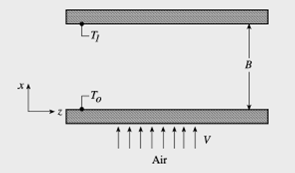a) Show that the inventory rate equation for energy becomes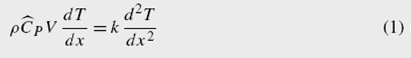b) Show that the introduction of the dimensionless variables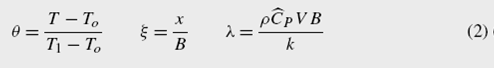reduces Eq. (1) to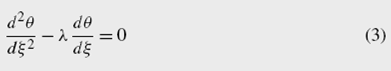c) Solve Eq. (3) and show that the velocity distribution is given as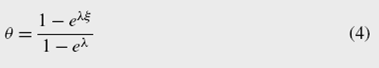d)Show that the heat flux at the lower plate is given by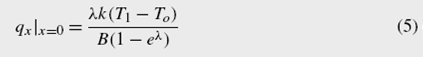View less »

Jul 25 2020 View more View LessSubscribe To Get Solution